﻿ 基于STM32和CAN总线的全向移动平台控制系统设计 Design of Omnidirectional Mobile Platform Control System Based on STM32 CAN Bus Control

Computer Science and Application
Vol.07 No.09(2017), Article ID:22169,8 pages
10.12677/CSA.2017.79102

Design of Omnidirectional Mobile Platform Control System Based on STM32 CAN Bus Control

Siqi Peng, Linxiao Tian, Yan Wang, Ming Liao, Zhilan Xiao, Kehua Zhang*

College of Engineering, Zhejiang Normal University, Jinhua Zhejiang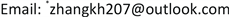Received: Sep. 10th, 2017; accepted: Sep. 23rd, 2017; published: Sep. 27th, 2017ABSTRACT

Omnidirectional mobile platform is driven by independent multiple Mecanum wheels. Through the combination of rotation speed and steering of the wheel set, it can move in a two-dimensional plane at arbitrary angles. Its movement is very flexible and smooth. It implements precise positioning and high precision trajectory control with fine adjustment of position, and is widely used in autonomous mobile robots. To improve the real-time and accuracy of omnidirectional mobile platform and enhance the stability and accuracy of the whole control system, the CAN bus network used in the platform, DMA data transmission, PID control and other methods were studied. Firstly, the mathematical modeling and motion analysis of the mobile platform based on the Mecanum wheel is carried out. Then, to speed up the transmission of sensor data, the platform uses DMA data transmission, and data exchange between main control and motor through CAN bus network is carried out at the speed of 1 M/S. Through repeated debugging and testing, operation is smooth during omnidirectional movement of the remote-control platform. And the platform without lag phenomenon, can quickly and accurately reach the target position. It basically meets the requirements of stable, reliable, high precision and strong anti-interference ability of the control system.

Keywords:Omnidirectional Mobile Platform, CAN Bus Network, DMA, PID AlgorithmCopyright © 2017 by authors and Hans Publishers Inc.

This work is licensed under the Creative Commons Attribution International License (CC BY).

http://creativecommons.org/licenses/by/4.0/1. 引言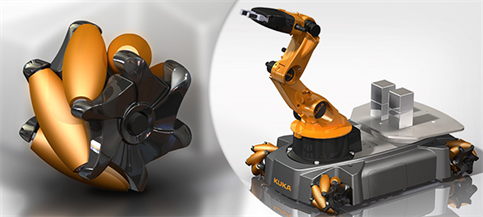Figure 1. Application of omnidirectional mobile platform

2. 移动平台系统的构成及运动分析

2.1. 全向移动平台系统的硬件构成

2.2. 移动平台运动分析

${v}_{{O}_{1}x}={v}_{x}-{w}_{0}{L}_{1}$ (1)

${v}_{{O}_{1}y}={v}_{y}-{w}_{0}{L}_{2}$ (2)

${v}_{{O}_{1}x}=-{v}_{g1}\mathrm{cos}\alpha +{w}_{1}R$ (3)

${v}_{{O}_{1}y}={v}_{g1}\mathrm{sin}\alpha$ (4)

$\left\{\begin{array}{l}{v}_{x}-{w}_{0}{L}_{1}=-{v}_{g1}\mathrm{cos}\alpha +{w}_{1}R\\ {v}_{y}-{w}_{0}{L}_{2}=-{v}_{g1}\mathrm{sin}\alpha \end{array}$ (5)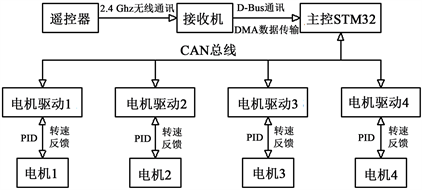Figure 2. Frame structure schematic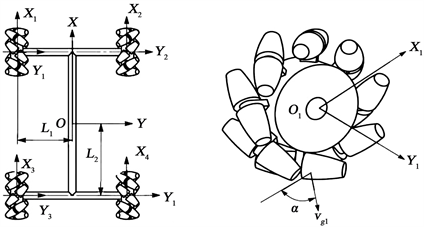Figure 3. Body coordinate system and 1st wheel coordinate system

${w}_{1}=\frac{1}{R}\left[\begin{array}{ccc}1& \frac{1}{\mathrm{tan}\alpha }& -\left({L}_{1}+\frac{{L}_{2}}{\mathrm{tan}\alpha }\right)\end{array}\right]\left[\begin{array}{c}{v}_{x}\\ {v}_{y}\\ {w}_{0}\end{array}\right]$ (6)

${w}_{1}=\frac{1}{R}\left[\begin{array}{ccc}1& 1& -\left({L}_{1}+{L}_{2}\right)\end{array}\right]\left[\begin{array}{c}{v}_{x}\\ {v}_{y}\\ {w}_{0}\end{array}\right]$ (7)

$\left[\begin{array}{c}{w}_{1}\\ {w}_{2}\\ {w}_{3}\\ {w}_{4}\end{array}\right]=\frac{1}{R}\left[\begin{array}{ccc}1& 1& -\left({L}_{1}+{L}_{2}\right)\\ 1& -1& \left({L}_{1}+{L}_{2}\right)\\ 1& -1& -\left({L}_{1}+{L}_{2}\right)\\ 1& 1& \left({L}_{1}+{L}_{2}\right)\end{array}\right]\left[\begin{array}{c}{v}_{x}\\ {v}_{y}\\ {w}_{0}\end{array}\right]$ (8)

3. 全向移动平台的控制系统

3.1. 软件设计思想与整体构架

3.2. CAN总线实现

CAN总线的全称是Control Area Network，属于现场总线的范畴，它是一种有效支持分布式控制或实时控制的串行通信网络。最早是由德国BOSCH公司设计用来解决汽车通信问题。解决了简单的点对点式通信使得电控系统电气连接线长度和接头数增加的问题，同时还提升了电控系统的稳定性和可维护性  。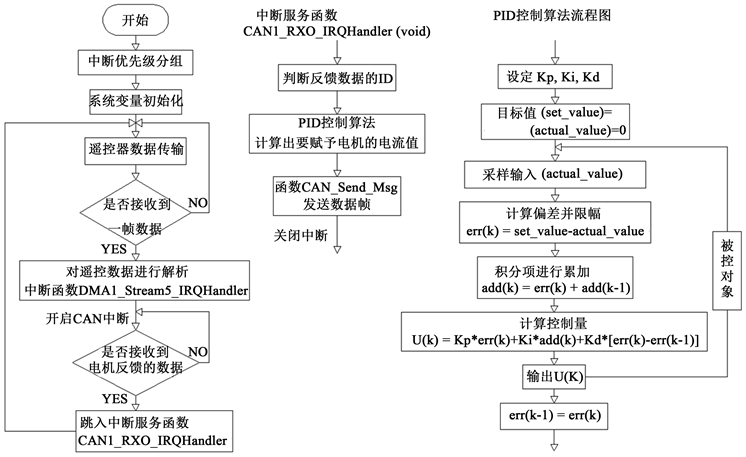Figure 4. Software flow chart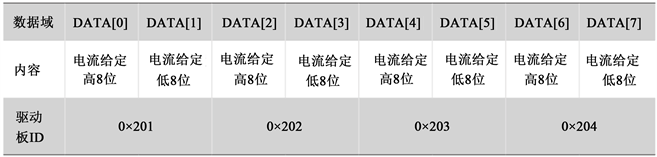Figure 5. The message format received by driver board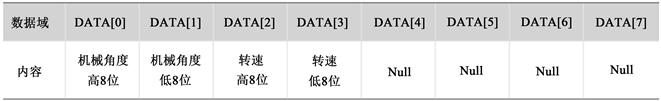Figure 6. The feedback message format from driver board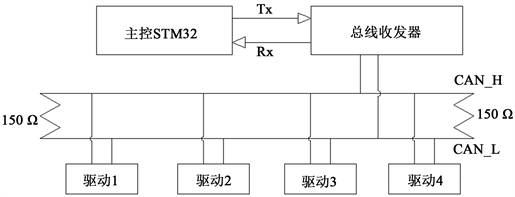Figure 7. CAN network hardware connection diagram

3.3. 遥控数据的DMA传输

DMA传输：全称是Direct Memory Access，直接通过存储器进行数据访问。该方法最大特点是无需CPU干预，也没有中断处理方式那样保留现场和恢复现场的过程，在硬件上搭建了一条RAM与I/O传输数据的快捷通道，很大程度减轻CPU的工作负载。CPU仅参与DMA初始化工作，而在整个数据传输过程中完全由DMA自身实现  。STM32芯片DMA资源丰富，我们选择DMA1的数据流5，4号通道。外设基地址为串口寄存器“USART2- > DR”，内存地址为自定义的数组Buffer[]。首先串口通过DMA控制器向主控发出DMA数据请求，主控响应DMA请求，把总线控制权交给DMA控制器，之后执行DMA数据传输，操作结束后DMA控制器把总线控制权交还给主控。数据从外设传输到内存中，每当遥控接收机向主控发送了一帧数据后，系统便触发中断，对遥控数据进行解析。

3.4. PID控制算法

PID控制器即P (比例)，I (积分)，D (微分)控制。它是对给定值 $r\left(t\right)$ 与实际输出值 $y\left(t\right)$ 的偏差 $err\left(t\right)$ 进行比例、积分、微分运算。它的数学表达式：

$U\left(t\right)=Kp\cdot \left(err\left(t\right)+\frac{1}{{T}_{I}}\cdot \underset{0}{\overset{t}{\int }}err\left(\tau \right)\text{d}\tau +{T}_{D}\cdot \frac{\text{d}err\left(t\right)}{\text{d}t}\right)$ (9)

$U\left(k\right)=Kp\cdot err\left(k\right)+Kp\cdot \frac{T}{{T}_{I}}\cdot \underset{1}{\overset{k}{\sum }}err\left(j\right)+Kp\cdot {T}_{d}\cdot \left(\frac{err\left(k\right)-err\left(k-1\right)}{T}\right)$

4. 移动平台测试

5. 结束语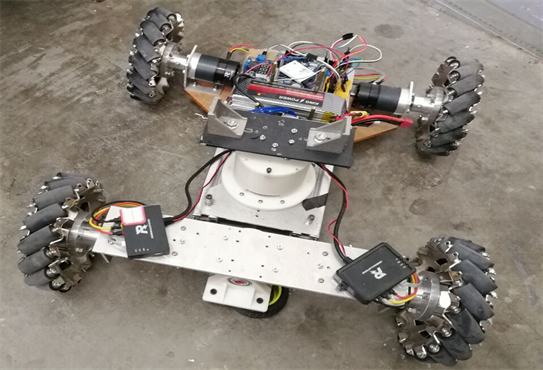Figure 8. Omnidirectional mobile test platform

Design of Omnidirectional Mobile Platform Control System Based on STM32 CAN Bus Control[J]. 计算机科学与应用, 2017, 07(09): 894-901. http://dx.doi.org/10.12677/CSA.2017.79102

1. 1. 肖建. 移动机器人分布式控制系统的研究与实现[D]: [硕士学位论文]. 广州: 暨南大学出版社, 2015.

2. 2. 田青. Mecanum轮式全向机器人位置精准控制的应用[D]: [硕士学位论文]. 南京: 东南大学出版社, 2015.

3. 3. 王兴松. Mecanum轮全方位移动机器人技术及其应用[J]. 机械制造与自动化, 2014, 43(3): 1-6.

4. 4. 陈博翁, 范传康, 贺骥. 基于麦克纳姆轮的全方位移动平台关键技术研究[J]. 东方电气评论, 2013, 27(108): 7-11.

5. 5. 柴文峰, 丁学明. 基于STM32单片机CAN通信控制网络设计[J]. 电子科技, 2017, 30(3): 142-145.

6. 6. 魏琳, 田波. 基于STM32F4系列的串口DMA数据处理传输研究[J]. 自动化应用, 2016(8): 92-93.

7. 7. 王虎, 彭如恕, 尹泉. 基于STM32嵌入式模糊PID步进电机控制系统的设计[J]. 机械工程师, 2014(11): 139-140.

8. NOTES



*通讯作者。